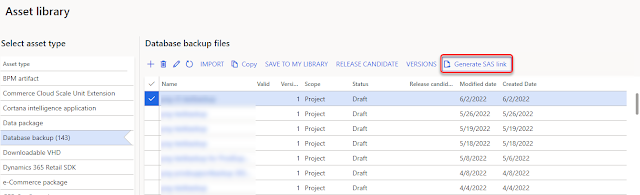## Monday, May 18, 2015

### Conditional IIF Sum in SSRS report at group level

I got a requirement where I want to show sum amount based on differnent conditions.

Simple Sum of amount can be shown with following expression;
=Sum(Fields!Amount.Value)

I tried following expression to show conditional sum on a group level; It did not work :(
=Sum(IIF(Fields!name.value = "Standard", Fields!Amount.value, 0))

I tried following expression after getting idea from this blog and it worked
=Sum(VAL(IIF(Fields!name.value = "Standard", Fields!Amount.value, 0)))

1.Thank you very Much

2.Great!It fixed my Result. Bravo!

3.4.Works like a charm! Thanks